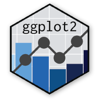Show Sidebar Hide Sidebar# geom_line in ggplot2

How to make line plots in ggplot2 with geom_line. Examples with code and interactive charts

### New to Plotly?

Plotly's R library is free and open source!
You can set up Plotly to work in online or offline mode.
We also have a quick-reference cheatsheet (new!) to help you get started!

### Version Check

Version 4 of Plotly's R package is now available!
Check out this post for more information on breaking changes and new features available in this version.

library(plotly)
packageVersion('plotly')

##  '4.9.1'


### Basic Line Plot

library(plotly)

dat1 <- data.frame(
sex = factor(c("Female","Female","Male","Male")),
time = factor(c("Lunch","Dinner","Lunch","Dinner"), levels=c("Lunch","Dinner")),
total_bill = c(13.53, 16.81, 16.24, 17.42)
)

p <- ggplot(data=dat1, aes(x=time, y=total_bill, group=sex)) +
geom_line() +
geom_point()

p <- ggplotly(p)

p


library(plotly)

dat1 <- data.frame(
sex = factor(c("Female","Female","Male","Male")),
time = factor(c("Lunch","Dinner","Lunch","Dinner"), levels=c("Lunch","Dinner")),
total_bill = c(13.53, 16.81, 16.24, 17.42)
)

# Map sex to different point shape, and use larger points
p <- ggplot(data=dat1, aes(x=time, y=total_bill, group=sex, shape=sex)) +
geom_line() +
geom_point()

p <- ggplotly(p)

p


### Styles & Themes

library(plotly)

dat1 <- data.frame(
sex = factor(c("Female","Female","Male","Male")),
time = factor(c("Lunch","Dinner","Lunch","Dinner"), levels=c("Lunch","Dinner")),
total_bill = c(13.53, 16.81, 16.24, 17.42)
)

p <- ggplot(data=dat1, aes(x=time, y=total_bill, group=sex, shape=sex, colour=sex)) +
geom_line(aes(linetype=sex), size=1) +     # Set linetype by sex
geom_point(size=5) +         # Use larger points, fill with white
scale_colour_hue(name="Sex",      # Set legend title
l=30)  +                  # Use darker colors (lightness=30)
scale_shape_manual(name="Sex",
values=c(22,21)) +      # Use points with a fill color
scale_linetype_discrete(name="Sex") +
xlab("Time of day") + ylab("Total bill") + # Set axis labels
ggtitle("Average bill for 2 people") +     # Set title
theme_bw()

p <- ggplotly(p)

p


### Continuous

library(plotly)

supp dose length
OJ  0.5  13.23
OJ  1.0  22.70
OJ  2.0  26.06
VC  0.5   7.98
VC  1.0  16.77
VC  2.0  26.14
')

p <- ggplot(data=datn, aes(x=dose, y=length, group=supp, colour=supp)) +
geom_line() +
geom_point()

p <- ggplotly(p)

p


### Categorical

library(plotly)

supp dose length
OJ  0.5  13.23
OJ  1.0  22.70
OJ  2.0  26.06
VC  0.5   7.98
VC  1.0  16.77
VC  2.0  26.14
')

datn2 <- datn
datn2$dose <- factor(datn2$dose)
p <- ggplot(data=datn2, aes(x=dose, y=length, group=supp, colour=supp)) +
geom_line() +
geom_point()

p <- ggplotly(p)

p


### Multiple Variables

library(reshape2)
library(plotly)

test_data <-
data.frame(
var0 = 100 + c(0, cumsum(runif(49, -20, 20))),
var1 = 150 + c(0, cumsum(runif(49, -10, 10))),
date = seq(as.Date("2002-01-01"), by="1 month", length.out=100)
)

test_data_long <- melt(test_data, id="date")  # convert to long format

p <- ggplot(data=test_data_long,
aes(x=date, y=value, colour=variable)) +
geom_line()

p <- ggplotly(p)

p


### Mulitple Points

library(plotly)
library(data.table)

d=data.table(x=seq(0, 100, by=0.1), y=seq(0,1000))
p <- ggplot(d, aes(x=x, y=y))+geom_line()
#Change the length parameter for fewer or more points
thinned <- floor(seq(from=1,to=dim(d),length=70))
p <- ggplot(d, aes(x=x, y=y))+geom_line()+geom_point(data=d[thinned,],aes(x=x,y=y))

p <- ggplotly(p)

p


### Styled Lines

library(plotly)

x <- c(10, 20, 50, 10, 20, 50)
mean = c(52.4, 98.2, 97.9, 74.1, 98.1, 97.6)
group = c(1, 1, 1, 2,2,2)
upper = c(13.64, 89, 86.4, 13.64, 89, 86.4)
lower = c(95.4, 99.8, 99.7, 95.4, 99.8, 99.7)
data <- data.frame(x=x,y=mean, group, upper, lower)

p <- ggplot(data, aes(x = x, y= mean, group = as.factor(data$group), colour=as.factor(data$group))) +
geom_line() + geom_point() +
geom_line(aes(y=lower),linetype="dotted") +
geom_line(aes(y=upper),linetype="dotted")+
scale_color_manual(name="Groups",values=c("red", "blue"))+
guides(colour = guide_legend(override.aes = list(linetype = 1)))

p <- ggplotly(p)

p


### Mapping to Groups

library(plotly)

# Data frame with two continuous variables and two factors
set.seed(0)
x <- rep(1:10, 4)
y <- c(rep(1:10, 2)+rnorm(20)/5, rep(6:15, 2) + rnorm(20)/5)
treatment <- gl(2, 20, 40, labels=letters[1:2])
replicate <- gl(2, 10, 40)
d <- data.frame(x=x, y=y, treatment=treatment, replicate=replicate)

p <- ggplot(d, aes(x=x, y=y, colour=treatment, group=interaction(treatment, replicate))) +
geom_point() + geom_line()

p <- ggplotly(p)

p


library(plotly)

x <- rep(1:10, 2)
y <- c(1:10, 1:10+5)
fac <- gl(2, 10)
df <- data.frame(x=x, y=y, fac=fac)

p <- ggplot(df, aes(x=x, y=y, linetype=fac)) +
geom_line() +
geom_segment(aes(x=2, y=7, xend=7, yend=7), colour="red") +
scale_linetype_discrete(guide=guide_legend(override.aes=aes(colour="blue")))

p <- ggplotly(p)

p


library(plotly)

# sample data
df <- data.frame(condition = rep(LETTERS[1:4], each = 5),
E = rep(1:5, times = 4),
avg = rnorm(20),
se = .3)
# plotting command
p <- ggplot(data = df, aes(x = E,
y = avg,
color = condition,
linetype = condition,
shape = condition,
fill = condition)) +
geom_line(size=1) +
geom_point(size=3) +
scale_color_manual(values = c(A = "red", B = "red", C = "blue", D = "blue"),
guide = "none") +
scale_linetype_manual(values = c(A = "solid", B = "dashed", C = "solid", D = "dashed"),
guide = "none") +
scale_shape_manual(values = c(A = 24, B = 24, C = 21, D = 21),
guide = "none") +
scale_fill_manual(values = c(A = "white", B = "red", C = "white", D = "blue"),
guide = "none") +
geom_errorbar(aes(x = E, ymin = avg-se, ymax = avg+se, color = NULL, linetype = NULL),
width=.1, position=position_dodge(width = .1))

p <- ggplotly(p)

p